# Topological Approach for Minimization of Cogging Torque in Permanent Magnet Synchronous Motors

Topological Approach for Minimization of Cogging Torque in Permanent Magnet Synchronous Motors
Robert Bosch GmbH, Electrical Drives, Buehl 77815, Germany

Faculty of Electrical- and Computer Engineering, Ilmenau 98693, Germany

Corresponding Author Email:
Page:
97-104
|
DOI:
https://doi.org/10.18280/ejee.220202
10 January 2020
|
Accepted:
8 March 2020
|
Published:
30 April 2020
| Citation

OPEN ACCESS

Abstract:

The purpose of this study is to find rotor structures of permanent magnet synchronous motors (PMSM) with very low cogging torque, while keeping the manufacturability feasible, preserving operating performance and demagnetization strength. Proposed topological approach divides the search space into discrete cells and distributes proper material into them using genetic algorithm. For better optimization efficiency, this method detects connected material areas, smooths their boundaries and retains original structure. The calculated performance curve takes into account an optimal current over the complete speed range. The obtained design shows significant improvements of the cogging torque behavior without being inferior in performance and demagnetization strength.

Keywords:

topology, optimization, rotor, structure, on/off approach, PMSM, cogging torque

1. Introduction

A large number of high-performance applications, demands smoothness of the torque as an important requirement. Presented paper shows reduction of the cogging torque in a Permanent Magnet Synchronous Motor (PMSM) using the topological method. This approach optimizes simultaneously the dimension, shape and structure of the used rotor in an automated way. Skewing and cancelling of the torque components by injecting of current harmonics are not a subject of this paper. Certain number of different topology optimization approaches can be found in the literature. Dyck introduces an optimum material distribution method . Buyun proposes a topology optimization using mutual energy and sensitivity analysis . Im proposes hybrid GA, ON/OFF sensitivity method . Wang considered as first magnetization directions within a topological optimization . Iles compares experience based and topological optimization methods . Kim introduces 3D topological optimization method . Choi introduced a topology optimization method using ON/OFF method concerning structural problems . Takahashi uses topological optimization to design implemented permanent magnet synchronous machine (IPMSM) using the ON/OFF method considering non-linear materials and rotation . Ishikawa proposes a topological optimization method using genetic algorithms, supported by cleaning function and building of material clusters . Sato introduces a multimaterial topology optimization with normalized Gaussian networks . Watanebe introduces ON/OFF topology optimization using immune algorithm . Ruzbehi  optimizes an electromagnetic actuator using a topology optimization and recognizes a neighbor’s in a post-processing step. An objective comparison of the different methods is still missing due to their low maturity level. Thus, choice of the proper algorithms remains specific to the actual problem. Deterministic and heuristic methods have been considered until now. Advantage of the deterministic methods is the ability to reach the optima faster than heuristic. On the other hand, heuristic approaches are more suitable for the search of global optima. Further, if choosing the ON/OFF method combined with evolutionary algorithms, input parameters can be represented easily in 2D encoding technique . Interpretation of the results is very clear without doubts about undefined states. Topology optimization is very different from the state of the art approach. This method is based on a division of the optimization domain into cells into which, best available material has to be placed. Designers work is limited to the definition of the objective function, boundaries, materials and optimization domain. Purpose of this paper is to improve significantly cogging torque behaviour of a permanent magnet electric machine, without losing machine performance. In order to achieve this goal, authors suggest an optimization of the rotor structure by an ON/OFF approach, supported by finite element approach and genetic algorithms. In order to obtain producible rotor designs exploring of the neighbourhood, grouping of the cells containing the same materials and smoothing the boundary shapes of found groups by an average builder is introduced. Using this technique, the checker board will be transformed into a more efficient model. Even sharp edges and small geometry details will be described accurately without significant increase of mesh elements. Further, in order to obtain smooth shapes without floating materials cells, algorithm for retention of the shape is proposed. A magnetization direction of the magnet material is specified within the model input and does not require additional elements, which would extend the optimization time. Additionally, the optimal current control for complete torque speed range based on analytic equations and unsaturated inductances is introduced, as well as an algorithm for the consideration of the demagnetization strength.

2. Proposed Optimization Approach

The approach starts with the division of the search space into a certain number of cells. Their number depends to the chosen resolution. In this work, one rotor pole pitch is divided into 1920 cells, 80 into tangential and 24 into radial direction as shown in Figure 1.

For the description of such model, input parameter was defined in Table 1 and illustrated in Figure 2. Parameter, rotor radius middle, allows different discretization of the design space close to the air gap since dense modelling of this area gives better cogging torque and torque pulsation results. Next step of the process is to decode and distribute the materials into the cells of the design space.

## 1.jpg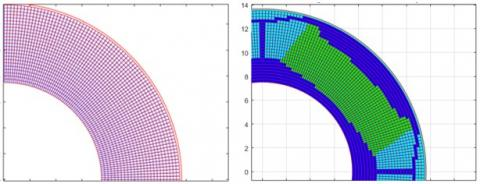(a)                                              (b)

Figure 1. Geometry from the discretized design space without placed materials (left) and with placed materials (right)

Table 1. Units for model description

## 2.jpg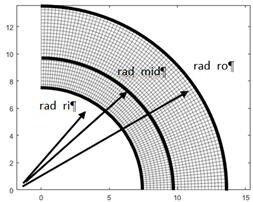Figure 2. Division of the design space into empty cells (80 tangential and 24 radial elements per pole pitch)

## 3.jpg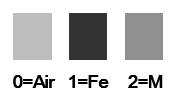Figure 3. Material encoding

In this work, “0” states an absence of the material or air, “1” stands for iron and “2” for permanent magnet material as “as described in Figure 3. Information decoded in that way is stored in the input vector mat_grid as shown in Table 1.

In comparison to the reference , where two magnetization elements were used, radial and tangential, in this work each cell has its own predefined magnetization orientation. Magnetization will get active only if the optimizer places permanent magnet material into it. An example for the radial orientation of the magnetization is shown in Figure 4a. Orientation of the magnetization in parallel and tangential directions to the magnet direct axis are shown in Figure 4b.

## 4.png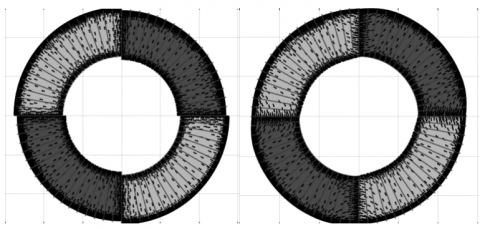(a)                                 (b)

Figure 4. Coercive field strength vectors for a four pole motor denoted by black arrows a) for radial magnetization direction b) for parallel and tangential magnetization direction. The zones are specified to be 20 deg/ 50 deg / 20 deg

Using this technique the size of the search space remains independent from the number of directions. In this case, radial and tangential magnetization directions are covered with an encoding pattern using three elements. Further, three magnetization zones per pole are available. Their absolute angles can be freely specified.

2.1 Material grouping and smoothing

Establishing of the groups follow after materials are assigned to all cells. A certain number of bordering cells with equal properties build groups.

Their determination is performed using flood fill algorithm , which is a very well-known technique from digital image processing. Figure 5 shows a domain with distributed materials 0, 1 and 2 and Figure 6 with recognized groups (4, 5, …, 13).

A pole pitch of a real finite element model of a 6 slot 4 pole permanent magnet synchronous motor before and after grouping of material cells is shown in Figure 7.

## 5.jpg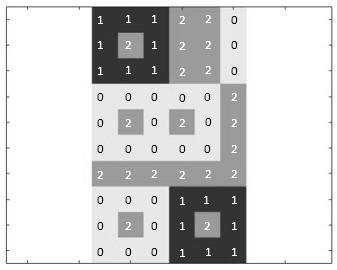Figure 5. Domain divided into cells with distributed material values (0, 1, 2)

## 6.jpg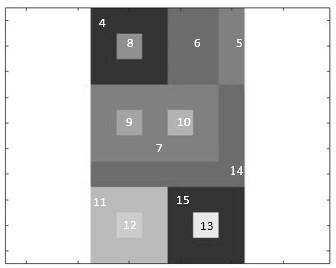Figure 6. Domain with detected material groups (group4 - group15) after using flood fill algorithm

## 7.png(a)                                              (b)

Figure 7. a) Meshed pole pitch of a 6 slot, 4 pole motor before and b) after grouping by flood fill approach

Thus, both motors have the same genetic constitution, after grouping the number of mesh elements is reduced by more than 50%. This will lead to significantly faster design evaluation. The structure of the rotor parts remains unchanged.

For the comparison before and after grouping, both models were calculated. Obtained results of back electromotive force (bEMF) are almost identical as shown in Figure 8. Whereas comparing the cogging torque, minor differences can be observed. This relationship is shown in Figure 9.

Those differences can be reduced by refining the mesh of the grouped model. Increase of the simulation time would increase slightly.

## 8.jpg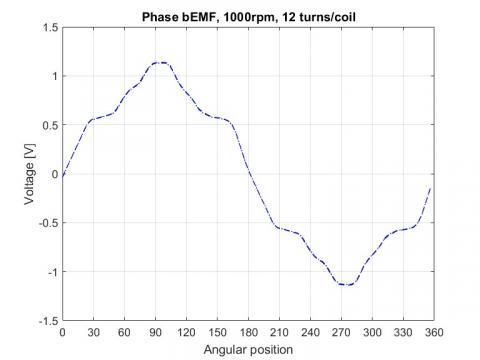Figure 8. Back electro motive force (bEMF) and of the 6 slot, 4 pole motor. Dotted line shows the curves of bEMF and before grouping and dot-dashed line after grouping by flood fill approach

## 9.jpg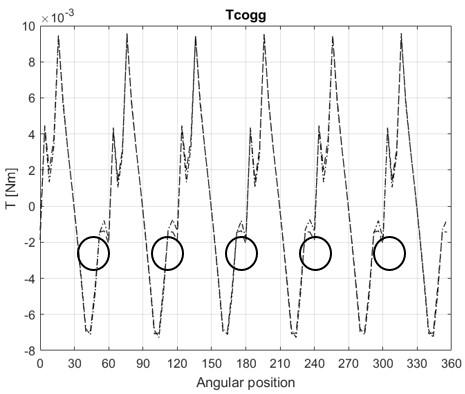Figure 9. Cogging torque of the 6 slot, 4 pole motor is shown. Dotted line shows the curves of bEMF and cogging torque before grouping and dot-dashed line after grouping by flood fill approach. Differences in cogging torque are marked by a circle

## 10.jpgFigure 10. Smoothing ($F_{s i}$) and restoring force ($F_{r i}$) applied on nodes. They are described in (1) and (2)

On the other hand, higher discretization of the gridded model would automatically lead into high increase of the simulation time. In order to evaluate cogging torque accurately, a high quality model is required. Usually the description of a small radii or curves is very inefficient with the grid model. In this work smoothing of the rotor component shapes is done by applying the smoothing ($\overrightarrow{F_{s l}}$) and restoring ($\overrightarrow{F_{r l}}$) forces to every node of the contour with additional building of an average value as shown in Figure 10. Equations for the smoothing and restoring force are given in (1) and (2).

$\overrightarrow{F_{s l}}=(\overrightarrow{x_{l-1}}+\overrightarrow{x_{l+1}}) / 2-\overrightarrow{x_{l}}$  (1)

$\overrightarrow{F_{r_{l}}}=(\overrightarrow{p_{l}}-\overrightarrow{x_{l}}) \cdot L$ (2)

with

L – weighting factor

pi-1, pi, pi+1 – Coordinates of point i-1, i and i+1 before smoothing

xi-1, xi, xi+1 – Coordinates of point i-1, i and i+1 after smoothing

Written in vector form, forces can be expressed as following:

$\overrightarrow{F_{s}}=0.5 \cdot A \cdot \vec{x}$  (3)

$\overrightarrow{F_{r}}=L \cdot(\vec{p}-\vec{x})$  (4)

with sparse matrix

$A=\left[\begin{array}{ccccc}2 & 1 & 0 & \cdots & 1 \\ 1 & 2 & 1 & \cdots & 0 \\ 0 & 1 & 2 & \cdots & 0 \\ \vdots & \vdots & \vdots & \ddots & \vdots \\ 1 & 0 & 0 & \cdots & 2\end{array}\right]$  (5)

Introducing weighting factor L, degree of smoothing gets adjustable as shown in Figure 11.

## 11.png(a)                                    (b)

Figure 11. Smoothed contour for a) L = 0.1 and b) L=1.0

Solving the force equilibrium (Fs=Fr), Eq. (6) is obtained.

$\vec{x}=E \cdot[\overrightarrow{p_{0}}]^{-1}$  (6)

with

$E=(0.5 \cdot A+I)$  (7)

and

$I=\left[\begin{array}{ccccc}1 & 0 & 0 & \cdots & 0 \\ 0 & 1 & 0 & \cdots & 0 \\ 0 & 0 & 1 & \cdots & 0 \\ \vdots & \vdots & \vdots & \ddots & \vdots \\ 0 & 0 & 0 & \cdots & 1\end{array}\right]$  (8)

2.2 Shape retaining for better manufacturability and plausibility check

Compared to experience based design, topological approach offers an additional degree of freedom. Exploring it, structures with higher performance can be found. However, found designs could be far away from being producible as shown in Figure 12. Considering available manufacturing processes, compromises have to be done. In order to exclude complex rotor parts from optimization process, an additional boundary condition is introduced.

In this paper, it is called shape retention. The aim of this measure is to restrict the sampling process, which has to prevent building of implausible magnet- and iron pieces or holes within the optimization domain. Sampling of the areas close to the group borders is focused. This measure is realized by the introduction of the mutation probability for each cell as shown in (9).

## 12.png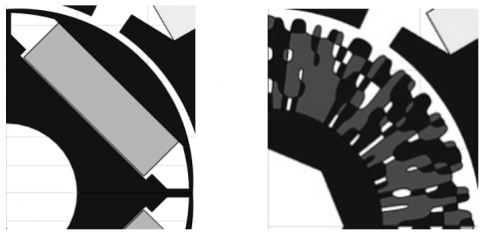(a)                                              (b)

Figure 12. Rotor structures a) Reference design b) Non-manufacturable design with improved cogging torque behaviour

## 13.jpg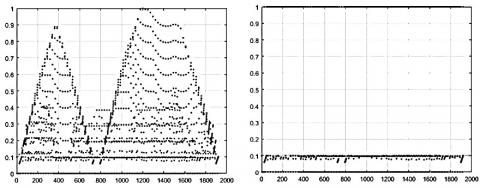(a)                                              (b)

Figure 13. P.u distance of each cell within optimization domain to the next border vs. index of the element a) Before manipulation b) after manipulation. Specified limit is set to 0.1 p.u.

$\overrightarrow{P_{g e s}}=\left[\begin{array}{ccc}P_{p o s(1,1)} & \cdots & P_{p o s(1, n)} \\ \vdots & \ddots & \vdots \\ P_{p o s(m, 1)} & \cdots & P_{p o s(m, n)}\end{array}\right] \cdot \overrightarrow{P_{b a s e}}$  (9)

with base mutation probability (Pbase). For their determination, minimal p.u. distances to the next order is calculated. Mutation probabilities of elements whose distance exceed the specified limit will be reduced as shown in Figure 13.

An example for sampling with and without manipulation of the probabilities is shown in Figure 14. At the end, the plausibility of the obtained structure has to be checked. If groups with less number of elements than specified are detected, design is stated to be invalid. In that case plausibility check process continues to sample new materials until all plausibility checks are fulfilled. Dependent to the level of defect high number of iterations is necessary. Nevertheless, processing time does not rise significantly since the FEM analysis is not necessary for this step.

## 14.png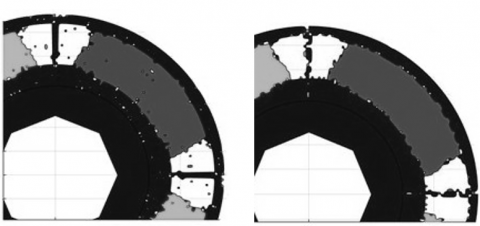(a)                                              (b)

Figure 14. Sampling of the search domain a) without using proposed manipulation b) and with using proposed manipulation

2.3 Shape retaining for better manufacturability and plausibility check

If the search of the optimum is in the beginning and the quality of the start design low, stochastic approach like genetic algorithm seem to be appropriate for the search of the global optimum . Further advantages like parallelization, clear interpretability of the results and intuitive and unproblematic implementation into existing tools or tool chains should be mentioned as well. Based on the mentioned criteria genetic algorithms combined with the ON/OFF approach for the structure optimization were chosen.

2.4 Optimal current control

Permanent magnet synchronous machines are usually used in variable speed drives operating in wide speed range. In order to ensure an optimal machine utilization, different control strategies are required . Below the base speed, maximum torque per ampere (MTPA) strategy is used.

## 15.jpg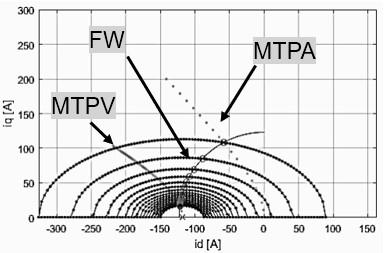Figure 15. Optimal control below and above base speed utilizing maximum torque per ampere (MTPA), field weakening (FW) and maximum torque per volt (MTPV)

Above the base speed field weakening (FW) is used and if feasible, maximum torque per volt (MTPV). Optimal direct (id) and quadrature axis current values (iq), for the reference design, are shown in Figure 15

2.5 Demagnetization strength

Used permanent magnets determine significantly the performance of the electrical machine. In the case of irreversible damage, typically by utilizing to high currents or exposing to high temperature, proper functionality cannot be fully guaranteed. As a result, topologically optimized machines must have comparable demagnetization properties as a reference. Comparable demagnetization strength definition is specified in (10).

$I_{\text {demag}} \geq I_{\text {ref}}-5 \%$  (10)

with

Idemag - demagnetization current of actual design

Iref    - demagnetization current of reference design

3. Simulation Results

The following case study considers a three phase PMSM which is appropriate for use in steering applications. The aim of the study is to decrease peak-to-peak cogging torque of the conventionally designed and optimized PMSM without losing performance at specified operating points or demagnetization strength. The Objective function and boundary conditions are listed in the Table 2.

Table 2. Objective function and boundary conditions

 Symbol Abbr. Quantity Unit SI Objective $O b j_{1}$ Min(Tcogg) Nm Boundary1 $B n d_{1}$ 1.4@3000rpm Nm Boundary2 $B n d_{2}$ 0,9@6000rpm rpm Boundary3 $B n d_{3}$ Demagnetization % Tot. Objective $O b j_{\text {tot}}$ - -

The objective function is defined in (11) and (12) and total objective function in (17) respectively. Boundary conditions are defined in (12) – (16). Violation of the specified boundary limits will not automatically exclude the particular design from the solution set but it will have strong negative impact. The impact can be scaled using weighting factors.

$O b j_{1}=\frac{1}{T_{cogg_{-r e l}}} \cdot f_{1}$  (11)

with weighting factor (f1)

$T_{cog g_{-} r e l}=\frac{T_{cog g_{-} a b s}}{T_{cog g_{-} r e f}}$  (12)

$B n d_{1}=\left\{\begin{array}{ll}T_{\text {rel@} 3000} & \text {if } T_{\text {rel@} 3000}<1 \\ 1 & \text {if } T_{\text {rel@} 3000} \geq 1\end{array}\right.$  (13)

$B n d_{2}=\left\{\begin{array}{ll}T_{r e l @ 6000} & \text { if } T_{r e l @ 6000}<1 \\ 1 & \text { if } T_{r e l @ 6000} \geq 1\end{array}\right.$  (14)

with p.u. mean torque ($T_{r e l}$)

$T_{r e l}=\frac{T_{a b s}}{T_{r e f}}$  (15)

and

$B n d_{3}=\left\{\begin{array}{lll}0 & \text { if } & \psi_{\text {loss}}<0.05 \\ f_{1} \cdot \psi_{\text {loss}} / \psi_{\text {ref}}, \text {if } & \psi_{\text {loss}} \geq 0.05\end{array}\right.$  (16)

with flux loss ($\psi_{l o s s}$)

$O b j_{t o t}=O b j_{1}+B n d_{1}+B n d 2_{3}+B n d_{3}$  (17)

In this work, performed optimization includes all methods described in II. As already shown, the optimization domain is divided into 1920 cells. Geometry of the domain is specified in Table I. Start design is equivalent to motor developed using state of the art methods. Design calculation and evaluation consists of the three main steps. Firstly, determination of the remanence value of demagnetized magnets is performed. As shown in (10), it should be again stated that only small demagnetization values are practically possible. Secondly, using the new remanence, motor parameters (Ld, Lq and PsiPM) under no-load condition are determined using FE Method as shown in the researches [17, 18].

## 16a.jpg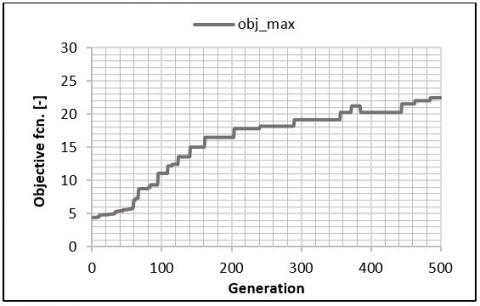(a)

## 16b.jpg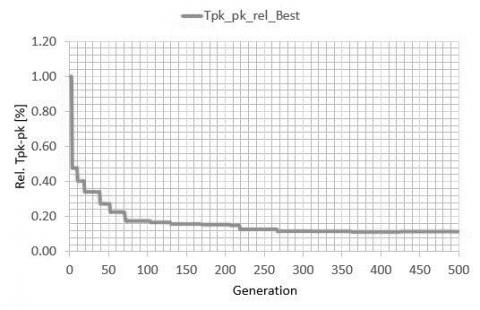(b)

Figure 16. Development of the a) total objective function b) the p.u. value of the cogging torque

Finally, optimal motor characteristic is calculated using mentioned methods in II. MTPA approach is used for speeds below base speed. Above base speed, FW and MTPV approaches are used. The optimization process converged after 500 generations (15000 FE calculations). Objective function increases by approx. 300% as shown in Fig. 16a. The peak-to-peak value of the cogging torque decreases by 90% as shown in Figure 16b. The no-load, back electro motive force (bEMF) and cogging torque of the reference and optimized machine are shown in Figure 17. The bEMF curves are as expected comparable. Cogging torque peak-to-peak value of the optimized design equals almost to zero.

## 17.jpgFigure 17. Back electromotive force (bEMF) and cogging torque of the reference and optimized design vs. angular rotor position

## 18.jpg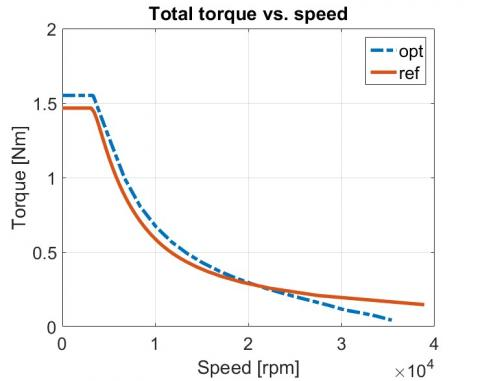Figure 18. Total torque of the reference and optimized design vs. angular position

## 19.jpg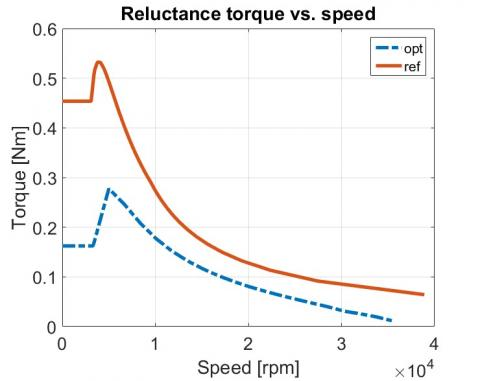Figure 19. Reluctance torque of the reference and optimized design vs. angular position

Achieved torque-speed characteristic of the optimized and reference design remain very similar. Both designs are fulfilling requirements in respect of torque, 1.5Nm at 3000rpm and 0.9 Nm at 6000 rpm. as shown in Figure 18.

The optimum trajectory changes, since ratio of the synchronous to reluctance torque decreases as shown in Figure 19. This can be also confirmed calculating the values of the direct (Ld) and quadrature inductances (Lq). For both, reference and optimum design, they are $L_{d}$ is equal to 43 / 42 µH and $L_{q}$ is equal 80 / 62 µH respectively. Their differences ($L_{d}-L_{q}$) are 37 / 20µH.

Further, it can be observed that after the optimization process, rotor bridges are missing. Further, notable is the unusual shape of the magnet as shown in Figure 20, which is significantly responsible for the reduction of the cogging torque level.

## 20.png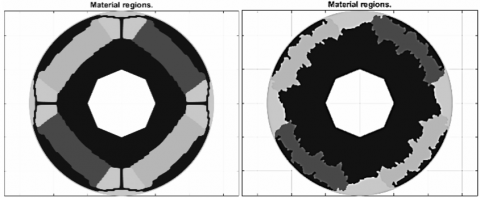(a)                                            (b)

Figure 20. Rotor cross sections of a) reference design b) optimized design

4. Conclusions

The multimaterial topology optimization approach with the grouping of the cells, additional smoothing and shape-preserving algorithm has been proposed. The main goal of this study was to find rotor design with significantly reduced cogging torque without worsening the performance of the machine. It has been shown that the proposed algorithm is able to find rotor designs with significantly improved cogging torque using low smoothing factor (L=0.01). Performed optimization led to a design with highly reduced cogging torque (reduction of 90%). The obtained motor did not show any significant disadvantage respectively to the defined requirements. At defined speeds, demanded torques could be reached. Shape and peak value of the electromotive force is comparable to that of the reference design. The demagnetization strength is slightly lower (-4%).

Compared to the design from Figure 12, which was obtained by performing the optimization without any additional rules, the manufacturability of the obtained design was highly improved. Nevertheless, some challenges still need to be overcome. If manufacturing by a grinding is considered, proposed magnet shapes should be smoother. This could be done by investigating the cogging torque with increased restoring force ($\overrightarrow{F_{r_{l}}}$). Alternatively, injection molding could be an interesting alternative for manufacturing. Since, the objective function was constructed to reduce the cogging torque and maximize the power of the electrical machine, optimal design does not contain iron bridges any more. This was also reflected in the decreased quadrature inductance. Further, considering the manufacturability, missing iron bridges complicate the fixing of the iron peace’s placed above the magnets. In order to overcome this issue, mandatory iron bridges, could be introduced as a further boundary condition.

Nomenclature
 GA Genetic algorithm PMSM Permanent Magnet Synchronous Machine IPMSM bMEF Implemented Permanent Magnet Synchronous Machine Back Electromotive Force, V Tcogg Cogging torque, Nm Trel Tabs Reluctance torque, Nm Absolute, mean torque, Nm Tref Cogging torque of reference design, Nm Idemag Demagnetization current, A Iref Demagnetization current of reference design, A Ld Direct axis Inductance, H Lq Quadrature axis Inductance, H PsiPM No-load, Permanent Magnet Flux, Vs MTPA Maximum Torque per Ampere FW Field Weakening MTPV Maximum Torque per Volt Greek symbols $\Psi_{l o s s}$ Flux loss due to the demagnetization, Vs $\Psi_{r e f}$ Flux loss of the reference design, Vs
References

 Dyck, D.N., Lowther, D.A. (1996). Automated design of magnetic devices by optimizing material distribution. IEEE Transactions on Magnetics, 32(3): 1181-1193. http://dx.doi.org/10.1109/20.497456

 Byun, J.K., Hahn, S.Y., Park, H. (1999). Topology optimization of electrical devices using mutual energy and sensitivity. IEEE Transactions on Magnetics, 35(5): 3718-3720. https://doi.org/10.1109/20.800642

 Im, C.H., Jun, H.K., Kim, Y.J. (2003). Hybrid genetic algorithms for electromangnetic topology optimization. IEEE Transactions on Magnetics, 39(5): 2163-2169. https://doi.org/10.1109/TMAG.2003.817094

 Wang, S., Youn, D., Moon, H., Kang, J. (2005). Topology optimization of electromagnetic systems considering magnetization direction. IEEE Transactions on Magnetics, 41(5): 1808-1811. https://doi.org/10.1109/TMAG.2005.846480

 Iles, D., Risticevic, M., Boldea, I. (2005). Advanced optimization design techniques for automotive interior permanent magnet synchronous machines. IEEE International Conference on Electric Machines and Drives, San Antonio, TX, USA, pp. 227-234. https://doi.org/10.1109/IEMDC.2005.195728

 Kim, D.H., Skykulski, J.K., Lowther, D.A. (2009). The implications of the use of composite materials in electromagnetics device topology and shape optimization. IEEE Transactions on Magnetics, 45(3): 1154-1157. http://dx.doi.org/10.1109/TMAG.2009.2012661

 Choi, J.S., Yoo, J. (2009). Structural topology optimization of magnetic actuators using genetic algorithms and ON/OFF sensitivity. IEEE Transactions on Magnetics, 45(5): 2276-2279. https://doi.org/10.1109/TMAG.2009.2016297

 Takahashi, N., Yamada, T., Miyagi, D. (2010). Examination of optimal design of IPM motor using ON/OFF method. IEEE Transactions on Magnetics, 46(8): 3149-3152. http://dx.doi.org/10.1109/TMAG.2010.2044382

 Ishikawa, T., Nakayama, K. (2012). Topology Optimization of rotor structure in brushless DC motor with concentrated windings using genetic algorithm combined with cluster of material. IEEE Transactions on Magnetics, 48(2): 899-902. http://dx.doi.org/10.1109/TMAG.2011.2176470

 Sato, T., Watanebe, K. (2015). Multimaterial topology optimization of electric machines based on normalized gaussian network. IEEE Transactions on Magnetics, 51(3): 7202604. http://dx.doi.org/10.1109/TMAG.2014.2359972

 Watanabe, K., Suga, T., Kitabatake, S. (2018). Topology optimization based on the ON/OFF method for synchronous motor. IEEE Transactions on Magnetics, 54(3): 7201104. http://dx.doi.org/10.1109/TMAG.2017.2751653

 Ruzbehi, S., Hahn, I. (2020). Two-level topology optimization of an electromagnetic actuator based on genetic algorithm and neighbourhood method. 2020 IEEE International Conference on Industrial Technology (ICIT), Buenos Aires, Argentina, pp. 230-233. https://doi.org/10.1109/ICIT45562.2020.9067305

 Wang, S.Y., Tai, K. (2003). A bit – array representation GA for structural topology optimization. The 2003 Congress on Evolutionary Computation, 2003. CEC '03, Canberra, ACT, Australia, Australia, pp. 671-677. https://doi.org/10.1109/CEC.2003.1299640

 Law, G. (2013). Quantitavie comparison of flood fill and modified flood fill algorithms. International Journal of Computer Theory and Engineering, 5(3): 503-508. https://doi.org/10.7763/IJCTE.2013.V5.738

 Zames, G., Ajlouni, N.M., Ajlouni, N.M., Ajlouni, N.M., Holland, J.H., Hills, W.D., Goldberg, D.E. (1981). Genetic algorithms in search, optimization and machine learning. Information Technology Journal, 3(1): 301-302.

 Schröder, D. (2009). Elektrische Antriebe-Regelung von Antriebssystemen, (in German). 4. Auflage, Springer Vierweg.

 Reichert, K., Binder, A. (2000). Elektrische Maschinen und Antriebe – Auswahl, Auslegung und Dimensionierung. Kursunterlagen, VDE-Verlag.

 Reichert, K., Kulig, S. (2001). Elektrische Maschinen und Antriebe – Numerische Verfahren für die Auslegung und Simulation. Kursunterlagen, VDE-Verlag.